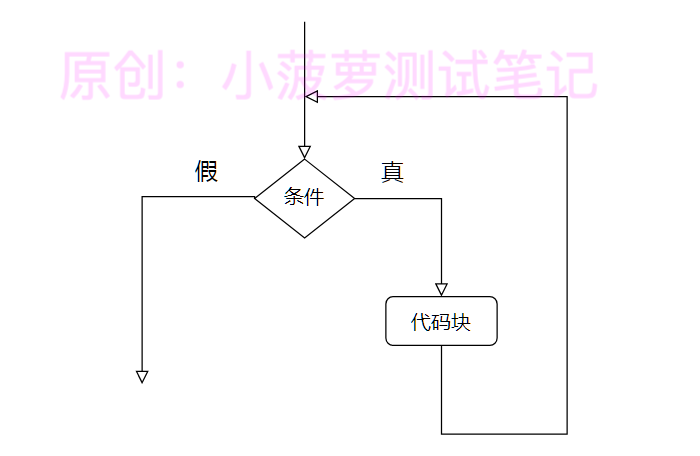# Python - while 循环

### 前言

• 在代码中有的时候我们需要程序不断地重复执行某一种操作
• 例如我们需要不停的判断某一列表中存放的数据是否大于 0，这个时候就需要使用循环控制语句
• 这里会讲解 while 循环

python 有两种循环语句，一个是 for、一个是 while

#### for 循环详解

https://www.cnblogs.com/poloyy/p/15087053.html

### while 循环语句

#### 循环结构1. 判断循环条件
2. 如果为真，则执行循环中的代码块；执行后跳转到步骤 1，重复第 1 步和第 2 步.
3. 如果为假，则循环结束

#### while 语法

while 条件:
代码块

#### 代码栗子

number = 1
while number <= 3:
print(number)
number += 1
print("结束循环")

# 输出结果
1
2
3

### break、continue 的详解

https://www.cnblogs.com/poloyy/p/15087598.html

### while + break 语句

• 这是一个检测 number 是否会素数的循环
• factor 是因子，通过循环取 2 到 number - 1 的数字
number = 9
is_prime = True
factor = 2
while factor < number:
print(factor)
# 如果可以整除因子，证明不是素数
if number % factor == 0:
is_prime = False
# 退出循环
break
# 循环自增
factor = factor + 1
print(is_prime)

# 输出结果
False

### while + continue 的栗子

# continue
sum = 0
number = 0
while number < 7:
number += 1
# 若是奇数则跳转到下一次循环
if number % 2 != 0:
continue
sum += number

# 求和
print(sum)

2+4+6

### while + else 的栗子

#### 语法格式

while 条件：
代码块 1
else:
代码块 2
• 当循环条件为真时，执行代码块 1
• 当循环条件为假时，执行代码块 2

#### 代码栗子一

# else
number = 1
while number <= 3:
print(number)
number = number + 1
else:
print('At the end:', number)

# 输出结果
1
2
3
At the end: 4

#### 代码栗子二

# else
number = 1
while number <= 5:
print(number)
number = number + 1
if number == 3:
# 提前结束循环
break
else:
print('At the end:', number)

# 输出结果
1
2

#### 重点

• 若想执行 else 里面的代码块，必须是触达到循环条件且为假
• 如果在循环里面提前结束了循环（break），则不会执行 else 里面的代码块

posted @ 2021-08-01 18:10  小菠萝测试笔记  阅读(252)  评论(0编辑  收藏  举报